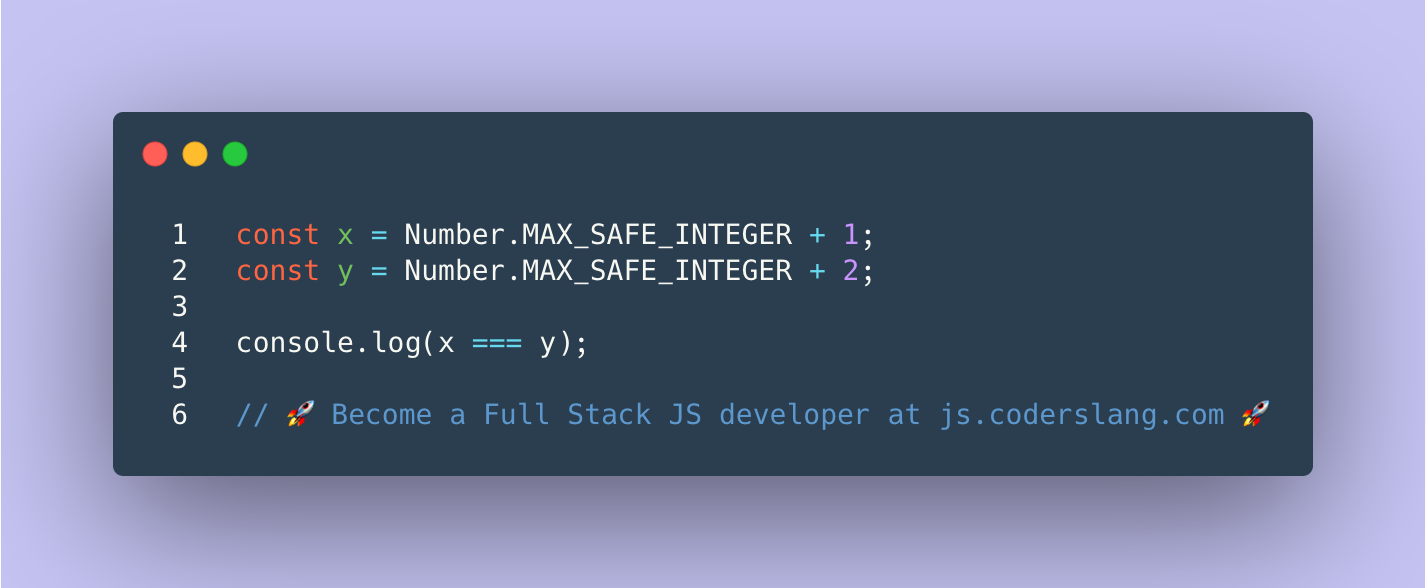True or false? What appears in the console?

.

.

.

.

.

.

.

.

.

.

.

.

.

.

.

.

.

JavaScript uses the double precision floating point numbers even to represent integers. This means that the biggest number that can be stored safely as a JS number is 2^53^ - 1 or 9007199254740991. This value is stored as a static constant `Math.MAX_SAFE_INTEGER`.

``````console.log(Math.MAX_SAFE_INTEGER);      // 9007199254740991
console.log(Math.pow(2, 53) - 1);        // 9007199254740991
``````

Having the value `Math.MAX_SAFE_INTEGER` doesn’t mean that it’s impossible to have a bigger number in JS. But, when we continue to increase the number, the loss of precision occurs.

``````console.log(Number.MAX_SAFE_INTEGER + 1); // 9007199254740992
console.log(Number.MAX_SAFE_INTEGER + 2); // 9007199254740992
``````

As you see, by adding 1 and then 2 to `Number.MAX_SAFE_INTEGER`, we got the same number.

If you’re building the application where such behavior is critical, then you should use `BigInt` instead of the regular JavaScript `Number`.

ANSWER: There will be a loss of precision due to rounding and safe integer overflow. Both `x` and `y` will equal `9007199254740992`. The message `true` will be printed to the console.